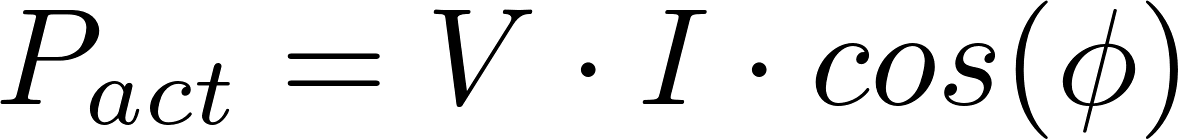# Power

Electric power is the rate at which energy is transferred by a circuit. The symbol for power is P and it is measured in watt (W). The Watt is defined as one joule per second. Electric power is equal to the voltage multiplied by the current.

### Formulas

The electric power can be calculated with one of the following formulas.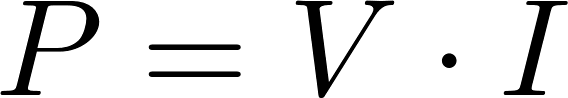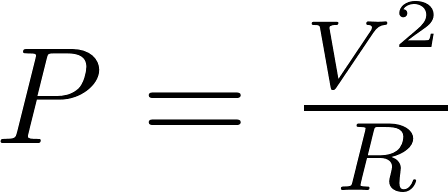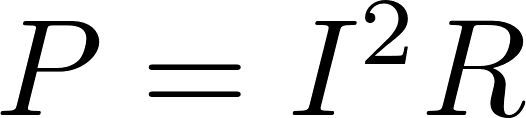P is the symbol for power and is measured in watt (W).
V is the symbol for voltage and is measured in volt (V).
I is the symbol for current and is measured in ampere (A).

### AC power

AC power

In an AC circuit, the voltage and current are varying, so the power can't be calculated with P = V·I. The (active) power can be calculated by multiplying the right side of the formula by cos(φ). cos(φ) is called the power factor and φ is the phase. The right side has to be multiplied with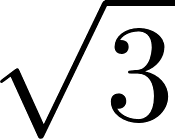when three phase power is used instead of one phase.

#### Formula